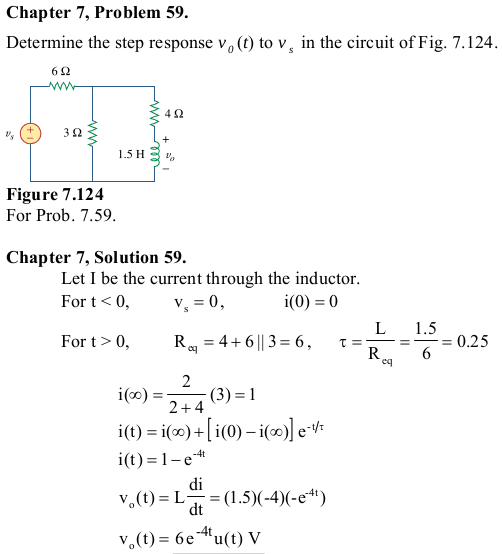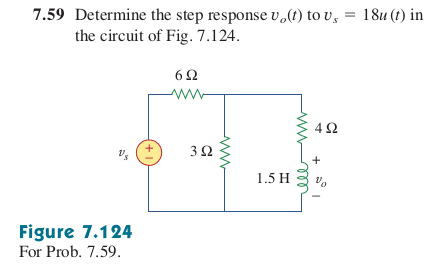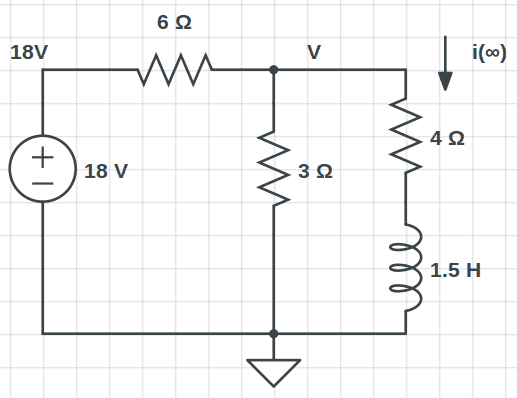# Finding the step response of RL circuit

• Engineering
Vishera

## Homework Statement## The Attempt at a Solution

I understand everything except how they got i(∞). It seems like they used current division on 3A. But where are they applying current division and where did they get 3A? Also, in the last step, they magically added u(t). Where did they get that from?

## Answers and Replies

Mentor
Looks like they failed to specify that the magnitude of the step for Vs was 18 V.

Vishera
Looks like they failed to specify that the magnitude of the step for Vs was 18 V.

Oh, opps. That was my mistake. It looks like the problem is slightly different in two places.

Here's the problem:But I still don't see where they got the 3A and where u(t) in the last step came from.

Mentor
Supposing that the source voltage will be 18 V for "a long time", what current be the steady state current provided by the source?

The u(t) specifies that the solution pertains to time t > 0 since the source is stepped from 0 V to 18 V according to 18u(t) V.

Homework Helper
Gold Member
You can write (18-v)/6 = v(1/3 + 1/4).
So 3 = v(1/6 + 1/3 + 1/4) = v(1/2 + 1/4)

So there's your 3A on the left ...

and i = v/4 = 3/(4/2 + 4/4) = 3*2/(4+2) = 1A for i(infinity).

Just how he managed to get to 3*2/(4+2) immediately I don't know.

Vishera
Supposing that the source voltage will be 18 V for "a long time", what current be the steady state current provided by the source?

The u(t) specifies that the solution pertains to time t > 0 since the source is stepped from 0 V to 18 V according to 18u(t) V.

Would the below be correct?$$\frac { v-18 }{ 6 } +\frac { v-0 }{ 3 } +\frac { v-0 }{ 4 } =0\\ v=4\\ \\ i(∞)=\frac { v-0 }{ 4 } =1\quad A$$

You can write (18-v)/6 = v(1/3 + 1/4).
So 3 = v(1/6 + 1/3 + 1/4) = v(1/2 + 1/4)

So there's your 3A on the left ...

I can see how you manipulated that equation to get 3A on the left side but I don't understand what the right side means. On the right side, we have a sum of 3 currents: v/6, v/3, and v/4. v/3 is the current from v to ground through the 3-ohm resistor. v/4 is the current from v to ground through the 4-ohm resistor. But what is v/6? It can't be the current from v to ground through the 6-ohm resistor because a 6-ohm resistor doesn't exist between v and ground. I just don't see where the 3A current is on the circuit.

Homework Helper
Gold Member
Would the below be correct?$$\frac { v-18 }{ 6 } +\frac { v-0 }{ 3 } +\frac { v-0 }{ 4 } =0\\ v=4\\ \\ i(∞)=\frac { v-0 }{ 4 } =1\quad A$$

I can see how you manipulated that equation to get 3A on the left side but I don't understand what the right side means. On the right side, we have a sum of 3 currents: v/6, v/3, and v/4. v/3 is the current from v to ground through the 3-ohm resistor. v/4 is the current from v to ground through the 4-ohm resistor. But what is v/6? It can't be the current from v to ground through the 6-ohm resistor because a 6-ohm resistor doesn't exist between v and ground. I just don't see where the 3A current is on the circuit.

You're looking for an actual 3A in the circuit where none exists. The 3A is just the result of rewriting my original equation which simply says that the current from the source to the v node = the sum of currents from the v node to ground.

Vishera
the current from the source to the v node = the sum of currents from the v node to ground.

I disagree here. On the right side, you had the term v/6 which is not a current from the v node to ground because there isn't a 6-ohm resistor between the v node and ground.

Homework Helper
Gold Member
I disagree here. On the right side, you had the term v/6 which is not a current from the v node to ground because there isn't a 6-ohm resistor between the v node and ground.

"I do not agree with what you have to say, but I'll defend to the death your right to say it."Voltaire